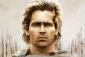# Time rates: hot air balloon rising straight up

2 posts / 0 new
Palarao JeffreyTime rates: hot air balloon rising straight up

a hot air balloon rising straight up from a level field is track by a range finder 500ft from the point of lift off. At the moment the lift finder's elevation is 45 degrees, the angle is increasing at the rate of 0.14 rad/min. How fast is the balloon rising/

Alexandertan theta = y/500
sec2 theta dtheta/dt = (1/500) dy/dt

When theta = 45 degrees
(1/cos2 45)(0.14) = (1/500) dy/dt
dy/dt = 140 ft/min

• Mathematics inside the configured delimiters is rendered by MathJax. The default math delimiters are $$...$$ and $...$ for displayed mathematics, and $...$ and $...$ for in-line mathematics.